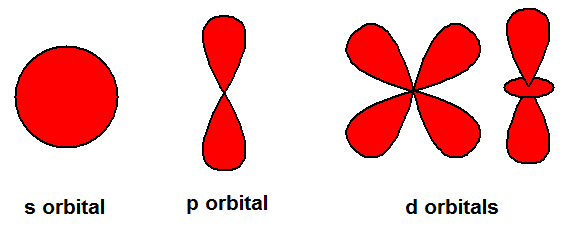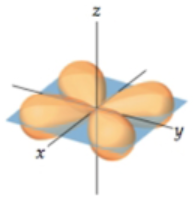# Problem: What is the azimuthal quantum number (also called the angular-momentum quantum number), ℓ, for the orbital shown here? Express your answer numerically as an integer.

###### FREE Expert Solution

angular momentum quantum number (ℓ) → (l) has to be at least 1 less than n, range of values from 0 up to (n-1)

▪ Each ℓ value corresponds to an orbital:

= 0 → s
= 1 → p
ℓ = 2 → d
ℓ = 3 → f

Recall the different shapes of the s, p, and d orbitals.86% (7 ratings)###### Problem Details

What is the azimuthal quantum number (also called the angular-momentum quantum number), ℓ, for the orbital shown here?